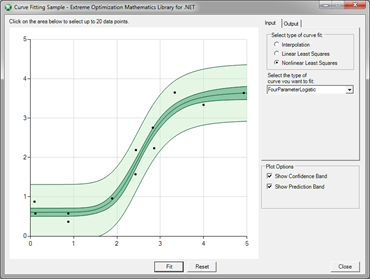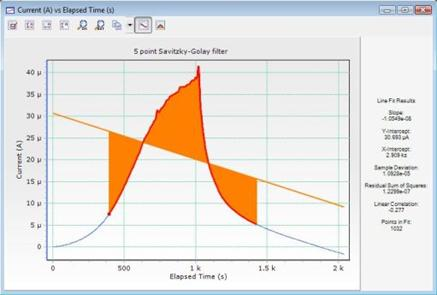Geeks With Blogs

# NET Mathematical Libraries

## .NET Builder for Matlab

• MATLAB Builder NE generates MATLAB based .NET and COM components
• royalty-free deployment
• creates the components by encrypting MATLAB functions and generating either a .NET or COM wrapper around them.

• a product that 2-way integrates Mathematica and Microsoft's .NET platform
• call .NET from Mathematica - use arbitrary .NET types directly from the Mathematica language.
• use and control the Mathematica kernel from a .NET program. turns Mathematica into a scripting shell to leverage the computational services of Mathematica.
• write custom front ends for Mathematica or use Mathematica as a computational engine for another program
• comes with full source code.
• Leverages MathLink - a Wolfram Research's protocol for sending data and commands back and forth between Mathematica and other programs. .NET/Link abstracts the low-level details of the MathLink C API.

## Extreme Optimization• a collection of general-purpose mathematical and statistical classes built for the.NET framework.
• It combines a math library, a vector and matrix library, and a statistics library in one package.
• Multi-core ready - Full support for Task Parallel Library features including cancellation.
• Broad base of algorithms covering a wide range of numerical techniques, including: linear algebra (BLAS and LAPACK routines), numerical analysis (integration and differentiation), equation solvers.
• Mathematics
• leverages parallelism using .NET 4.0's Task Parallel Library.
• Basic math: Complex numbers, 'special functions' like Gamma and Bessel functions, numerical differentiation.
• Solving equations: Solve equations in one variable, or solve systems of linear or nonlinear equations.
• Curve fitting: Linear and nonlinear curve fitting, cubic splines, polynomials, orthogonal polynomials.
• Optimization: find the minimum or maximum of a function in one or more variables, linear programming and mixed integer programming.
• Numerical integration: Compute integrals over finite or infinite intervals, over 2D and higher dimensional regions. Integrate systems of ordinary differential equations (ODE's).
• Fast Fourier Transforms: 1D and 2D FFT's using managed or fast native code (32 and 64 bit)
• BigInteger, BigRational, and BigFloat: Perform operations with arbitrary precision.
• Vector and Matrix Library
• Real and complex vectors and matrices.
• Single and double precision for elements.
• Structured matrix types: including triangular, symmetrical and band matrices.
• Sparse matrices.
• Matrix factorizations: LU decomposition, QR decomposition, singular value decomposition, Cholesky decomposition, eigenvalue decomposition.
• Portability and performance: Calculations can be done in 100% managed code, or in hand-optimized processor-specific native code (32 and 64 bit).
• Statistics
• Data manipulation: Sort and filter data, process missing values, remove outliers, etc. Supports .NET data binding.
• Statistical Models: Simple, multiple, nonlinear, logistic, Poisson regression. Generalized Linear Models. One and two-way ANOVA.
• Hypothesis Tests: 12 14 hypothesis tests, including the z-test, t-test, F-test, runs test, and more advanced tests, such as the Anderson-Darling test for normality, one and two-sample Kolmogorov-Smirnov test, and Levene's test for homogeneity of variances.
• Multivariate Statistics: K-means cluster analysis, hierarchical cluster analysis, principal component analysis (PCA), multivariate probability distributions.
• Statistical Distributions: 25 29 continuous and discrete statistical distributions, including uniform, Poisson, normal, lognormal, Weibull and Gumbel (extreme value) distributions.
• Random numbers: Random variates from any distribution, 4 high-quality random number generators, low discrepancy sequences, shufflers.
• New in version 4.0 (November, 2010)
• Support for .NET Framework Version 4.0 and Visual Studio 2010
• TPL Parallellized – multicore ready
• sparse linear program solver - can solve problems with more than 1 million variables.
• Mixed integer linear programming using a branch and bound algorithm.
• special functions: hypergeometric, Riemann zeta, elliptic integrals, Frensel functions, Dawson's integral.
• Full set of window functions for FFT's.
 Product Price Update subscription Single Developer License \$999 \$399 Team License (3 developers) \$1999 \$799 Department License (8 developers) \$3999 \$1599 Site License (Unlimited developers in one physical location) \$7999 \$3199

## NMath• matrix and vector classes
• random number generators
• Fast Fourier Transforms (FFTs)
• numerical integration
• linear programming
• linear regression
• curve and surface fitting
• optimization
• hypothesis tests
• analysis of variance (ANOVA)
• probability distributions
• principal component analysis
• cluster analysis
• built on the Intel Math Kernel Library (MKL), which contains highly-optimized, extensively-threaded versions of BLAS (Basic Linear Algebra Subroutines) and LAPACK (Linear Algebra PACKage).
 Product Price Update subscription Single Developer License \$1295 \$388 Team License (5 developers) \$5180 \$1554

## DotNumerics

• free
• DotNumerics is a website dedicated to numerical computing for .NET that includes a C# Numerical Library for .NET containing algorithms for Linear Algebra, Differential Equations and Optimization problems.
• The Linear Algebra library includes CSLapack, CSBlas and CSEispack, ports from Fortran to C# of LAPACK, BLAS and EISPACK, respectively.
• Linear Algebra (CSLapack, CSBlas and CSEispack). Systems of linear equations, eigenvalue problems, least-squares solutions of linear systems and singular value problems.
• Differential Equations. Initial-value problem for nonstiff and stiff ordinary differential equations ODEs (explicit Runge-Kutta, implicit Runge-Kutta, Gear's BDF and Adams-Moulton).
• Optimization. Unconstrained and bounded constrained optimization of multivariate functions (L-BFGS-B, Truncated Newton and Simplex methods).

## Math.NET Numerics

• free
• an open source numerical library - includes special functions, linear algebra, probability models, random numbers, interpolation, integral transforms.
• A merger of dnAnalytics with Math.NET Iridium
• in addition to a purely managed implementation will also support native hardware optimization.
• constants & special functions
• complex type support
• real and complex, dense and sparse linear algebra (with LU, QR, eigenvalues, ... decompositions)
• non-uniform probability distributions, multivariate distributions, sample generation
• alternative uniform random number generators
• descriptive statistics, including order statistics
• various interpolation methods, including barycentric approaches and splines
• numerical function integration (quadrature) routines
• integral transforms, like fourier transform (FFT) with arbitrary lengths support, and hartley
• spectral-space aware sequence manipulation (signal processing)
• combinatorics, polynomials, quaternions, basic number theory.
• parallelized where appropriate, to leverage multi-core and multi-processor systems
• fully managed or (if available) using native libraries (Intel MKL, ACMS, CUDA, FFTW)
• provides a native facade for F# developers

Related Posts on Geeks With Blogs Matching Categories

Comments on this post: NET Math Libraries

# re: NET Math LibrariesWe would like to evaluate this library for our website ipracticemath.com. We would like to know that does this library support symbolic algebra as this will help a lot in solving physics problems.

Thanks.
James
Left by prash on Sep 20, 2013 1:39 PM

# re: NET Math LibrariesThis is such a useful process in my recent project. - Antiquities of California
Left by Williams George on Dec 29, 2016 5:04 AM